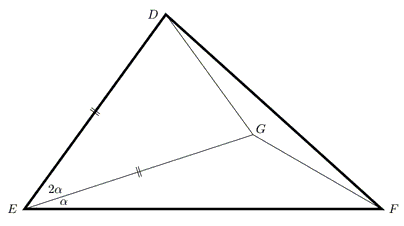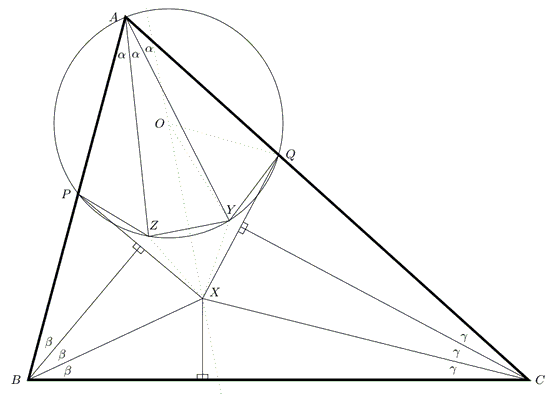Morley's Theorem

Morley's theorem asserts that in the diagram below $\Delta XYZ$ is equilateral, whatever $\Delta ABC.$What follows has been communicated to me in private correspondence by Roger Smyth. Roger has based his new direct proof on the following

Lemma

Assume point $G$ in the interior of $\Delta DEF,$ with $\angle FED = 3\alpha,$ satisfies $\angle FEG=\alpha$ and $DE=EG.$Then $DG=FG$ iff $\angle DFE$ equals $60^{\circ}-\alpha.$

Proof

Assume $DG=FG$ and let $H$ be the foot of the perpendicular from $G$ to $EF.$Since $GF = GD = 2GH$ it follows that $\angle GFE = 30^{\circ}.$ Thus, for the reflex $\angle DGF,$

\begin{align} \angle DGF &= \angle DGE +\angle EGH + \angle FGH \\ &= 2(90^{\circ}-\alpha) + 60^{\circ} \\ &= 240^{\circ} -2\alpha. \end{align}

Since we assumed $\Delta DGF$ isosceles, $\angle DFG =\angle FDG=30^{\circ}-\alpha;$ therefore, $\angle DFE=60^{\circ}-\alpha,$ as required.

Proof of Morley's TheoremAbove (as usual) $X$ is the Morley vertex adjacent to $BC$ and $P$ and $Q$ are the points on $AB$ and $AC$ such that $BP = BX$ and $CQ = CX.$ The circle through $A,$ $P,$ $Q$ is drawn and the angle trisectors from $A$ cut it at $Z$ and $Y.$ Thus the arcs $PZ,$ $ZY,$ $YQ$ are equal. Let the centre of the circle be $O.$ Since $\Delta XPQ$ is isosceles, the perpendicular bisector of $YZ$ goes through both $O$ and $X.$ Moreover, $\angle XOQ = 3\alpha$ and $\angle XOY = \alpha$ and $OY = OQ,$ so the conditions of the lemma are met in $\Delta QOX.$ $(Q \leftrightarrow D,$ $O \leftrightarrow E,$ $X \leftrightarrow F,$ $Y \leftrightarrow G).$

Now comes the crunch. The Morley point next to $A$C lies at the intersection of two trisectors, one of which is $AY$ and the other is also the perpendicular bisector of $QX.$ But $\angle QXO = \frac{1}{2}\angle QXP = 60^{\circ}-\alpha$ and, according to the lemma, this means that $QY=XY;$ thus the perpendicular bisector of $QX$ actually goes through $Y.$ So our search for the Morley point is over almost before it has begun! By symmetry $Z$ is the Morley vertex adjacent to $AB$ and $\Delta XYZ$ is equilateral because $PZ = ZX = XY = YQ = YZ.$Morley's Miracle

Invalid proofs